How Cheenta works to ensure student success?
Explore the Back-Story

# INMO 2013 Question No. 1 Solution1     Let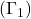and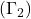be two circles touching each other externally at R. Letandbe the centres ofand, respectively. Let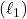be a line which is tangent toat P and passing through, and letbe the line tangent toat Q and passing through. Let. If KP=KQ then prove that the triangle PQR is equilateral.

Discussion:

We note that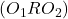is a straight line (why?)
Alsoare right angled triangles with right angles at point Q and P respectively.
Hence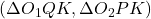are similar (vertically opposite angles and right angles)
Thus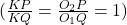as KP =KQ.
Hence the radii of the two circles are equal..
This implies R is the midpoint of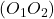hence the midpoint of hypotenuse of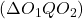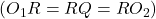since all are circum-radii of.
Henceis equilateral, similarly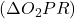is also equilateral.
Thusis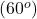also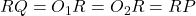.
Hence triangle PQR is equilateral.

1     Letandbe two circles touching each other externally at R. Letandbe the centres ofand, respectively. Letbe a line which is tangent toat P and passing through, and letbe the line tangent toat Q and passing through. Let. If KP=KQ then prove that the triangle PQR is equilateral.

Discussion:

We note thatis a straight line (why?)
Alsoare right angled triangles with right angles at point Q and P respectively.
Henceare similar (vertically opposite angles and right angles)
Thusas KP =KQ.
Hence the radii of the two circles are equal..
This implies R is the midpoint ofhence the midpoint of hypotenuse ofsince all are circum-radii of.
Henceis equilateral, similarlyis also equilateral.
Thusisalso.
Hence triangle PQR is equilateral.

This site uses Akismet to reduce spam. Learn how your comment data is processed.

### One comment on “INMO 2013 Question No. 1 Solution”

1.Anonymous says:

the solution to first question isn't complete...you will also hav to contradict the case where tangents are opposite

### Knowledge Partner